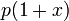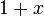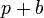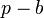# Effect of sales tax on market price and quantity traded

(diff) ← Older revision | Latest revision (diff) | Newer revision → (diff)

This article discusses the effect of a sales tax on the market price and equilibrium quantity traded for a good.

## Assumptions

Prior to beginning the analysis, we note the following:

1. A sales tax may be price-proportional (proportional to the price of the trade) or quantity-proportional (proportional to the quantity being traded). The quantitative analysis differs somewhat in both these cases. However, the qualitative analysis largely does not.
2. In this article, we largely focus on the effect of the introduction of a sales tax, by performing comparative statics between a world without sales tax and a world with sales tax. Much of this analysis can also be applied to increases in sales tax. Conversely, a decrease in, or elimination of, a sales tax should have the opposite effect.
3. For the most part, we focus on short run effects. In particular, this means that we assume the law of demand and law of supply.
4. We assume away the costs of compliance with the tax laws, and do not deal with issues of tax evasion.
5. For the most part, we assume competitive markets (though we also discuss other cases). Hence, the law of one price is assumed to hold, so that we can talk of the market price.

## What we are interested in tracking

In the world with no tax, there are two measures of interest:

We are interested in tracking three measures:

• The pre-tax market price, i.e., the effective price that the seller gets to keep.
• The post-tax market price, i.e., the effective price that the buyer pays. This is obtained by adding the sales tax to the pre-tax market price.

## Effect of sales tax on a single good or class of goods with a competitive market

We consider comparative statics between two situations:

• A world where there are no sales taxes
• A world where sales taxes are introduced on a single good (or class of goods) for which we are drawing the supply and demand curves.

Note that the same analysis also works for comparative statics where we change the sales tax on only one class of goods.

### Analytical tools

There are three kinds of diagrams that we draw to study the situation:

1. Consider the world without sales tax. We can draw the usual supply and demand curves and do the usual analysis to find the market price and equilibrium quantity traded.
2. Consider the world with sales tax. In this world, consider supply and demand curves drawn with respect to pre-tax prices.
3. Consider the world with sales tax. In this world, consider supply and demand curves with respect to post-tax prices.

### Analysis with pre-tax prices

We want to perform comparative statics between the world without sales tax and the world with sales tax. We consider the supply and demand curves for the latter in terms of the pre-tax prices.

Supply curve: The supply curve using pre-tax prices is expected to remain the same as the supply curve in a world without taxes, because the price that the seller sees is the pre-tax price.

Demand curve: The demand curve changes. In general, it moves downward. Assuming the law of demand, that is the same as moving inward, i.e., a contraction of the demand curve. Arithmetically, the new demand curve is related to the old demand curve as follows:

• For a price-proportional sales tax with a factor of$x$, the new demand function at a price of$p$ equals the old demand function at a price of$p(1 + x)$. The reason is that, as far as buyers are concerned, the effective price is$p(1 + x)$. The upshot is that the demand curve shrinks downward by a factor of$1 + x$.
• For a quantity-proportional sales tax with a tax of$b$ per unit quantity, the new demand function at a price of$p$ is the old demand function at a price of$p + b$. The reason is that, as far as buyers are concerned, the effective price is$p + b$. The upshot is that the demand curve shifts downward by a vertical distance of$b$.

The upshot is that the supply curve remains the same and the demand curve moves inward. Thus, as with the general analysis of comparative statics for demand and supply, we obtain that:

• The market price drops. In other words, the new pre-tax market price is lower than the market price in the world without taxes.
• The equilibrium quantity traded falls.

### Analysis with post-tax prices

We want to perform comparative statics between the world without sales tax and the world with sales tax. We consider the supply and demand curves for the latter in terms of the post-tax prices.

Demand curve: The demand curve using post-tax prices is expected to remain the same as the demand curve in a world without taxes, because the price that the buyer sees is the post-tax price.

Supply curve: The supply curve changes. In general, it moves upward. Assuming the law of supply, that is the same as moving inward, i.e., a contraction of the supply curve. Arithmetically, the new supply curve is related to the old supply curve as follows:

• For a price-proportional sales tax with a factor of$x$, the new supply function at a price of$p$ equals the old supply function at a price of$p/(1 + x)$. The reason is that, as far as sellers are concerned, the effective price is the pre-tax price$p/(1 + x)$. The upshot is that the supply curve moves upward by a factor of$1 + x$.
• For a quantity-proportional sales tax with a tax of$b$ per unit quantity, the new supply function at a price of$p$ is the old supply function at a price of$p - b$. The reason is that, as far as sellers are concerned, the effective price is$p - b$. The upshot is that the supply curve shifts upward by a vertical distance of$b$.

The upshot is that the supply curve contracts and the demand curve remains the same. Thus, as with the general analysis of comparative statics for demand and supply, we obtain that:

• The market price rises. In other words, the new ppost-tax market price is higher than the market price in the world without taxes.
• The equilibrium quantity traded falls.

### Combined analysis

Combining both these analyses, we obtain the three conclusions:

• The pre-tax market price is lower than the market price in a world without the tax
• The post-tax market price is higher than the market price in a world without the tax
• The equilibrium quantity traded is less than the equilibrium quantity traded in a world without the tax

### Extreme cases of elastic and inelastic supply and demand

We consider some extreme cases. The first row describes the standard case, and subsequent rows describe extreme cases:

Assumption for price-elasticity of demand Assumption for price-elasticity of supply Conclusion about pre-tax market price (relative to market price in a world without the tax) Conclusion about post-tax market price (relative to market price in a world without the tax) Conclusion about equilibrium quantity traded (relative to a world without the tax)
negative (satisfies the law of demand) positive (satisfies the law of supply) falls rises falls
infinite, i.e., a horizontal demand curve (e.g., when the good has a perfect substitute) positive (satisfies the law of supply) falls stays the same falls
zero, i.e., a vertical demand curve. We also say that the demand is perfectly price-inelastic positive (satisfies the law of supply) stays the same rises stays the same
negative (satisfies the law of demand) infinite, i.e., a horizontal supply curve, e.g., a constant cost industry stays the same rises falls
negative (satisfies the law of demand) zero, i.e., a vertical supply curve. We say that the supply is perfectly price-inelastic falls stays the same stays the same